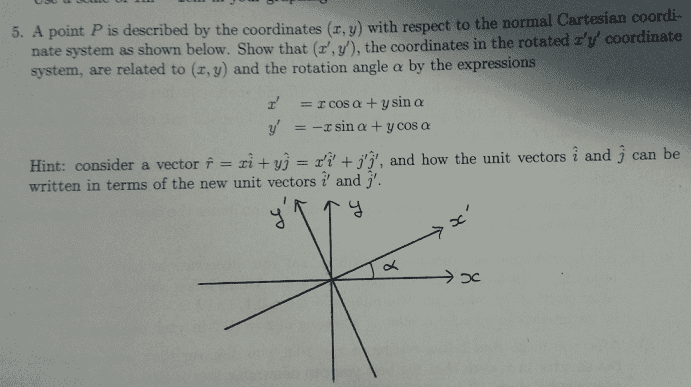# Wording of a question

Panphobia
Wording of a question (vectors)

## Homework Statement

I do not need help for a solution, I only need clarification on the question. I do not understand what the question is asking, here is a picture.What does it mean when it says the rotation angle alpha? Are x' and y' two different vectors? Also what am I supposed to try to prove? Also what does point P have to do with anything?

Last edited:

Homework Helper
Gold Member

## Homework Statement

I do not need help for a solution, I only need clarification on the question. I do not understand what the question is asking, here is a picture.What does it mean when it says the rotation angle alpha? Are x' and y' two different vectors? Also what am I supposed to try to prove? Also what does point P have to do with anything?

Alpha is the tilt angle of the primed system with respect to the unprimed system. x' and y' are the coordinates of the primed system just as x and y are the coordinates of the unprmed system.

It is suggested that you show how a point P in the unprimed system, being the same point in the primed system, can be written in the xy and the x'y' systems. Then equivalencies can be deduced relating the primed x' and y' to the unprimed x and y values.

Panphobia
So the question is saying to show that the coordinates (x,y) with respect to the normal Cartesian plane, are related to the different coordinates of that same point in the x',y' plane, with those equations. What is he exactly asking you to show? If he already gives you the solution. Is he asking your for the process in which you get those two expressions?

Last edited: